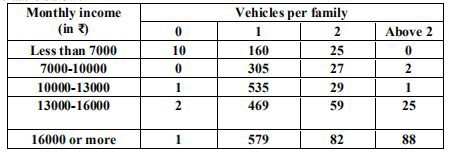# NCERT Solutions Class 9 Mathematics Solutions for Probability - Exercise 15.1 in Chapter 15 - Probability

. An organisation selected 2400 families at random and surveyed them to determine a relationship

between income level and the number of vehicles in a family. The information gathered is listed

in the table below:Suppose a family is chosen. Find the probability that the family chosen is

(i) earning ₹10000 – 13000 per month and owning exactly 2 vehicles.

(ii) earning ₹16000 or more per month and owning exactly 1 vehicle.

(iii) earning less than ₹7000 per month and does not own any vehicle.

(iv) earning ₹13000 – 16000 per month and owning more than 2 vehicles.

(v) owning not more than 1 vehicle.

Total number of families = 2400

(i) Numbers of families earning ₹10000 –13000 per month and owning exactly 2 vehicles = 29

∴, the probability that the family has chosen is earning ₹10000 – 13000 per month and owning

exactly 2 vehicle =\frac{29}{2400}

(ii) Number of families earning ₹16000 or more per month and owning exactly 1 vehicle = 579

∴, the probability that the family chosen is earning ₹16000 or more per month and owning

exactly 1 vehicle = =\frac{579}{2400}

(iii) Number of families earning less than ₹7000 per month and does not own any vehicle = 10

∴, the probability that the family chosen is earning less than ₹7000 per month and does not

own any vehicle =\frac{10}{2400}=\frac{1}{240}

(iv) Number of families earning ₹13000-16000 per month and owning more than 2 vehicles = 25

∴, the probability that the family chosen is earning ₹13000 – 16000 per month and owning

more than 2 vehicles = =\frac{25}{2400}=\frac{1}{96}

(v) Number of families owning not more than 1 vehicle = 10+160+0+305+1+535+2+469+1+579

= 2062

∴, the probability that the family chose owns not more than 1 vehicle =\frac{2062}{2400}=\frac{1031}{1200}

Related Questions
Exercises

Lido

Courses

Teachers

Book a Demo with us

Syllabus

Maths
CBSE
Maths
ICSE
Science
CBSE

Science
ICSE
English
CBSE
English
ICSE
Coding

Terms & Policies

Selina Question Bank

Maths
Physics
Biology

Allied Question Bank

Chemistry
Connect with us on social media!